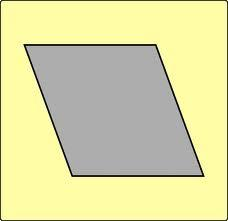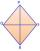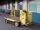# Diamond

Determine the side of diamond if its content is S = 353 cm2 and one diagonal u2 = 45 cm.

Correct result:

u1 =  15.7 cm

#### Solution:We would be pleased if you find an error in the word problem, spelling mistakes, or inaccuracies and send it to us. Thank you!#### You need to know the following knowledge to solve this word math problem:

We encourage you to watch this tutorial video on this math problem:

## Next similar math problems:

• DiamondCalculate the length of the two diagonals of the diamond if: a = 13 cm v = 12 cm
• Rhombus diagonalArea of rhombus is 224. One diagonal measures 33, find length of other diagonal.
• Two numbersWe have two numbers. Their sum is 140. One-fifth of the first number is equal to half the second number. Determine those unknown numbers.
• TreesA certain species of tree grows an average of 0.5 cm per week. Write an equation for the sequence that represents the weekly height of this tree in centimeters if the measurements begin when the tree is 200 centimeters tall.
• Volleyball8 girls wants to play volleyball against boys. On the field at one time can be six players per team. How many initial teams of this girls may trainer to choose?
• Two equationsSolve equations (use adding and subtracting of linear equations): -4x+11y=5 6x-11y=-5
• Factory and divisionsThe factory consists of three auxiliary divisions total 2,406 employees. The second division has 76 employees less than 1st division and 3rd division has 212 employees more than the 2nd. How many employees has each division?
• First manWhat is the likelihood of a random event where are five men and seven women first will leave the man?
• One halfOne half of ? is: ?
• TransformerTransformer - U1 = 230 V, N1 = 300, N2 = 1,200, I1 = 4 A. Calculate the transformation ratio, voltage and current in the secondary coil.
• Functions f,gFind g(1) if g(x) = 3x - x2 Find f(5) if f(x) = x + 1/2
• 6 termsFind the first six terms of the sequence. a1 = 7, an = an-1 + 6
• PowersExpress the expression ? as the n-th power of the base 10.
• Linear systemSolve a set of two equations of two unknowns: 1.5x+1.2y=0.6 0.8x-0.2y=2
• Nineteenth memberFind the nineteenth member of the arithmetic sequence: a1=33 d=5 find a19
• WheelchairThe boy pushes the wheelchair with a force of F1 = 20N. The wheelchair exerts a force of F2 on the boy. How large is this force?
• Percentage increaseIncrease number 400 by 3.5%